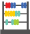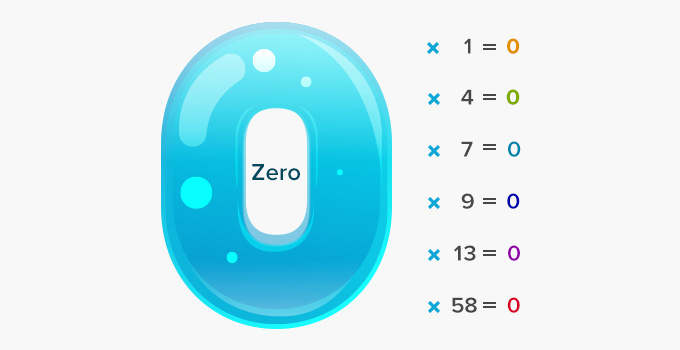# Zero property of multiplication - Definition with Examples

The Complete Prek-5 Learning Program Built for Your Child
Home > Zero property of multiplication

##Let's learn!

What is the zero property of multiplication?
According to the zero property of multiplication, the product of any number and zero, is zero.

Here are some examples of the zero property of multiplication.Fun Facts ZERO is the only number which has so many names such as nought, naught, nil, zilch and zip.

##Let's sing!

Multiplying numbers by zero is fun
The product is also zero; that is none!

##Let's do it!

Play a game with your child. Ask him/her to be as prompt as they can be while replying, when you ask them to multiply numbers. See if they answer correctly when you ask them to multiply big and small numbers by zero.

##Related math vocabulary